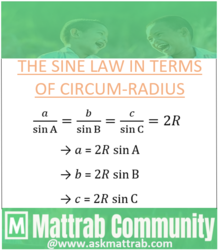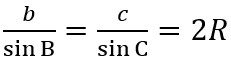The Sine Law in terms of Circum-RadiusLet ABC be the triangle with all three vertex on the circumference of the circle with center O as shown in fig above.
BO is drawn if the angle is either acute or obtuse as shown in fig(i) and fig(ii). Also, BO is  produced to D to form the diameter BD as shown in fig. [C coincides D in fig (iii)]
Then, circum-radius=OB=R(radius of circle) and BD=2R and angle BCD=900 [in fig (i) and (ii)] being angle at semi-circle.
To Prove:1. For a triange as shown in fig(i)
BAC=BDC                     [angle subtended by same segment]
i.e., angle A = angle D.........(i)
In triangle BDC,2. For a triangle as shown in fig(ii)In triangle BDC,3. For a triangle as shown in fig(iii)
BC = 2R = 2R sin A                    [A=900 ,﻿ sin A = sin 900 = 1]Hence, for every condition we have,Similarly, we can prove,Combining these we get,, which is the Sine Law in terms of circum-radius.